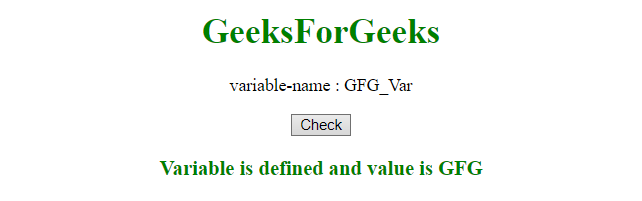# JavaScript | Check the existence of variable

JavaScript has a bulit-in function to check whether a variable is defined/initialized or undefined.

Note:

• The typeof operator will check whether a variable is defined or not.
• The typeof operator doesn’t throw a ReferenceError exception when it is used with an undeclared variable.
• The typeof null will return an object. So, check for null also.

Example 1: This example checks whether a variable is defined or not.

 `  ` `<``html``>  ` ` `  `<``head``>  ` `    ``<``title``>  ` `        ``JavaScript to check existence of variable ` `    `` ` `  ` `         `  `<``body` `style` `= ``"text-align:center;"``>  ` `     `  `    ``<``h1` `style` `= ``"color:green;"` `>  ` `        ``GeeksForGeeks  ` `    ``  ` `             `  `    ``<``p``> ` `        ``variable-name : GFG_Var ` `    `` ` ` `  `    ``<``button` `onclick``=``"myGeeks()"``>  ` `        ``Check ` `    ``  ` `     `  `    ``<``h3` `id` `= ``"div"` `style``=``"color:green;"``> ` `             `  `    ``` `    ``<``script``>  ` `        ``function myGeeks() { ` `             `  `            ``var GFG_Var; ` `            ``var h3 = document.getElementById("div"); ` `             `  `            ``if (typeof GFG_Var === 'undefined') { ` `                ``h3.innerHTML = "Variable is Undefined"; ` `            ``} ` `            ``else { ` `                ``h3.innerHTML = "Variable is defined and" ` `                    ``+ " value is " + GFG_Var; ` `            ``} ` `        ``} ` `    ``  ` `  ` ` `  `                     `

Output:

• Before clicking the button:• After clicking the button:Example 2: This example also checks whether a variable is defined or not.

 `  ` `<``html``>  ` ` `  `<``head``>  ` `    ``<``title``>  ` `        ``JavaScript to check existence of variable ` `    `` ` `  ` `         `  `<``body` `style` `= ``"text-align:center;"``>  ` `     `  `    ``<``h1` `style` `= ``"color:green;"` `>  ` `        ``GeeksForGeeks  ` `    ``  ` `             `  `    ``<``p``> ` `        ``variable-name : GFG_Var ` `    `` ` ` `  `    ``<``button` `onclick``=``"myGeeks()"``>  ` `        ``Check ` `    ``  ` `     `  `    ``<``h3` `id` `= ``"div"` `style``=``"color:green;"``> ` `             `  `    ``` `    ``<``script``>  ` `        ``function myGeeks() { ` `         `  `            ``var GFG_Var = "GFG"; ` `            ``var h3 = document.getElementById("div"); ` `             `  `            ``if (typeof GFG_Var === 'undefined') { ` `                ``h3.innerHTML = "Variable is Undefined"; ` `            ``} ` `            ``else { ` `                ``h3.innerHTML = "Variable is defined and" ` `                    ``+ " value is " + GFG_Var; ` `            ``} ` `        ``} ` `    ``  ` `  ` ` `  `                     `

Output:

• Before clicking the button:• After clicking the button:Example 3: The previous example doesn’t check for null value of variable. This example also checks a variable is null or not.

 `  ` `<``html``>  ` ` `  `<``head``>  ` `    ``<``title``>  ` `        ``JavaScript to check existence of variable ` `    `` ` `  ` `         `  `<``body` `style` `= ``"text-align:center;"``>  ` `     `  `    ``<``h1` `style` `= ``"color:green;"` `>  ` `        ``GeeksForGeeks  ` `    ``  ` `             `  `    ``<``p``> ` `        ``variable-name : GFG_Var ` `    `` ` ` `  `    ``<``button` `onclick``=``"myGeeks()"``>  ` `        ``Check ` `    ``  ` `     `  `    ``<``h3` `id` `= ``"div"` `style``=``"color:green;"``> ` `             `  `    ``` `    ``<``script``>  ` `        ``function myGeeks() { ` `      `  `            ``var GFG_Var = null; ` `            ``var h3 = document.getElementById("div"); ` `             `  `            ``if (typeof GFG_Var === 'undefined' || GFG_Var === null ) { ` `                ``h3.innerHTML = "Variable is Undefined"; ` `            ``} ` `            ``else { ` `                ``h3.innerHTML = "Variable is defined and value is " ` `                        ``+ GFG_Var; ` `            ``} ` `        ``} ` `    ``  ` `  ` ` `  `                     `

Output:

• Before clicking the button:• After clicking the button:My Personal Notes arrow_drop_upCheck out this Author's contributed articles.

If you like GeeksforGeeks and would like to contribute, you can also write an article using contribute.geeksforgeeks.org or mail your article to contribute@geeksforgeeks.org. See your article appearing on the GeeksforGeeks main page and help other Geeks.

Please Improve this article if you find anything incorrect by clicking on the "Improve Article" button below.